Inspired by recent progress on various enhanced versions of Transformer models, this post presents how the vanilla Transformer can be improved for longer-term attention span, less memory and computation consumption, RL task solving, etc.

It has been almost two years since my last post on attention. Recent progress on new and enhanced versions of Transformer motivates me to write another post on this specific topic, focusing on how the vanilla Transformer can be improved for longer-term attention span, less memory and computation consumption, RL task solving and more.

### Notations

Symbol Meaning
$d$ The model size / hidden state dimension / positional encoding size.
$h$ The number of heads in multi-head attention layer.
$L$ The segment length of input sequence.
$\mathbf{X} \in \mathbb{R}^{L \times d}$ The input sequence where each element has been mapped into an embedding vector of shape $d$, same as the model size.
$\mathbf{W}^k \in \mathbb{R}^{d \times d_k}$ The key weight matrix.
$\mathbf{W}^q \in \mathbb{R}^{d \times d_k}$ The query weight matrix.
$\mathbf{W}^v \in \mathbb{R}^{d \times d_v}$ The value weight matrix. Often we have $d_k = d_v = d$.
$\mathbf{W}^k_i, \mathbf{W}^q_i \in \mathbb{R}^{d \times d_k/h}; \mathbf{W}^v_i \in \mathbb{R}^{d \times d_v/h}$ The weight matrices per head.
$\mathbf{W}^o \in \mathbb{R}^{d_v \times d}$ The output weight matrix.
$\mathbf{Q} = \mathbf{X}\mathbf{W}^q \in \mathbb{R}^{L \times d_k}$ The query embedding inputs.
$\mathbf{K} = \mathbf{X}\mathbf{W}^k \in \mathbb{R}^{L \times d_k}$ The key embedding inputs.
$\mathbf{V} = \mathbf{X}\mathbf{W}^v \in \mathbb{R}^{L \times d_v}$ The value embedding inputs.
$S_i$ A collection of key positions for the $i$-th query $\mathbf{q}_i$ to attend to.
$\mathbf{A} \in \mathbb{R}^{L \times L}$ The self-attention matrix between a input sequence of lenght $L$ and itself. $\mathbf{A} = \text{softmax}(\mathbf{Q}\mathbf{K}^\top / \sqrt{d_k})$.
$a_{ij} \in \mathbf{A}$ The scalar attention score between query $\mathbf{q}_i$ and key $\mathbf{k}_j$.
$\mathbf{P} \in \mathbb{R}^{L \times d}$ position encoding matrix, where the $i$-th row $\mathbf{p}_i$ is the positional encoding for input $\mathbf{x}_i$.

## Attention and Self-Attention

Attention is a mechanism in the neural network that a model can learn to make predictions by selectively attending to a given set of data. The amount of attention is quantified by learned weights and thus the output is usually formed as a weighted average.

Self-attention is a type of attention mechanism where the model makes prediction for one part of a data sample using other parts of the observation about the same sample. Conceptually, it feels quite similar to non-local means. Also note that self-attention is permutation-invariant; in other words, it is an operation on sets.

There are various forms of attention / self-attention, Transformer (Vaswani et al., 2017) relies on the scaled dot-product attention: given a query matrix $\mathbf{Q}$, a key matrix $\mathbf{K}$ and a value matrix $\mathbf{V}$, the output is a weighted sum of the value vectors, where the weight assigned to each value slot is determined by the dot-product of the query with the corresponding key:

And for a query and a key vector $\mathbf{q}_i, \mathbf{k}_j \in \mathbb{R}^d$ (row vectors in query and key matrices), we have a scalar score:

where $S_i$ is a collection of key positions for the $i$-th query to attend to.

See my old post for other types of attention if interested.

The multi-head self-attention module is a key component in Transformer. Rather than only computing the attention once, the multi-head mechanism splits the inputs into smaller chunks and then computes the scaled dot-product attention over each subspace in parallel. The independent attention outputs are simply concatenated and linearly transformed into expected dimensions.

where $[.;.]$ is a concatenation operation. $\mathbf{W}^q_i, \mathbf{W}^k_i \in \mathbb{R}^{d \times d_k/h}, \mathbf{W}^v_i \in \mathbb{R}^{d \times d_v/h}$ are weight matrices to map input embeddings of size $L \times d$ into query, key and value matrices. And $\mathbf{W}^o \in \mathbb{R}^{d_v \times d}$ is the output linear transformation. All the weights should be learned during training.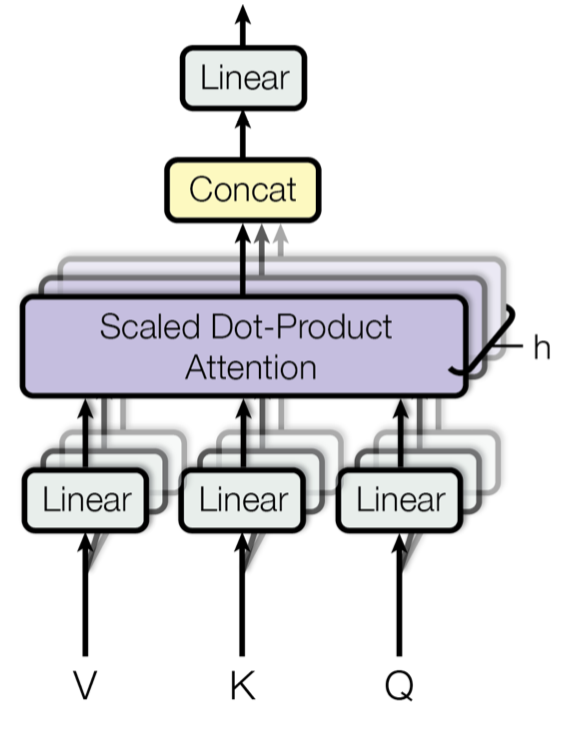Fig. 1. Illustration of the multi-head scaled dot-product attention mechanism. (Image source: Figure 2 in Vaswani, et al., 2017)

## Transformer

The Transformer (which will be referred to as “vanilla Transformer” to distinguish it from other enhanced versions; Vaswani, et al., 2017) model has an encoder-decoder architecture, as commonly used in many NMT models. Later decoder-only Transformer was shown to achieve great performance in language modeling tasks, like in GPT and BERT.

Encoder-Decoder Architecture

The encoder generates an attention-based representation with capability to locate a specific piece of information from a large context. It consists of a stack of 6 identity modules, each containing two submodules, a multi-head self-attention layer and a point-wise fully connected feed-forward network. By point-wise, it means that it applies the same linear transformation (with same weights) to each element in the sequence. This can also be viewed as a convolutional layer with filter size 1. Each submodule has a residual connection and layer normalization. All the submodules output data of the same dimension $d$.

The function of Transformer decoder is to retrieve information from the encoded representation. The architecture is quite similar to the encoder, except that the decoder contains two multi-head attention submodules instead of one in each identical repeating module. The first multi-head attention submodule is masked to prevent positions from attending to the future.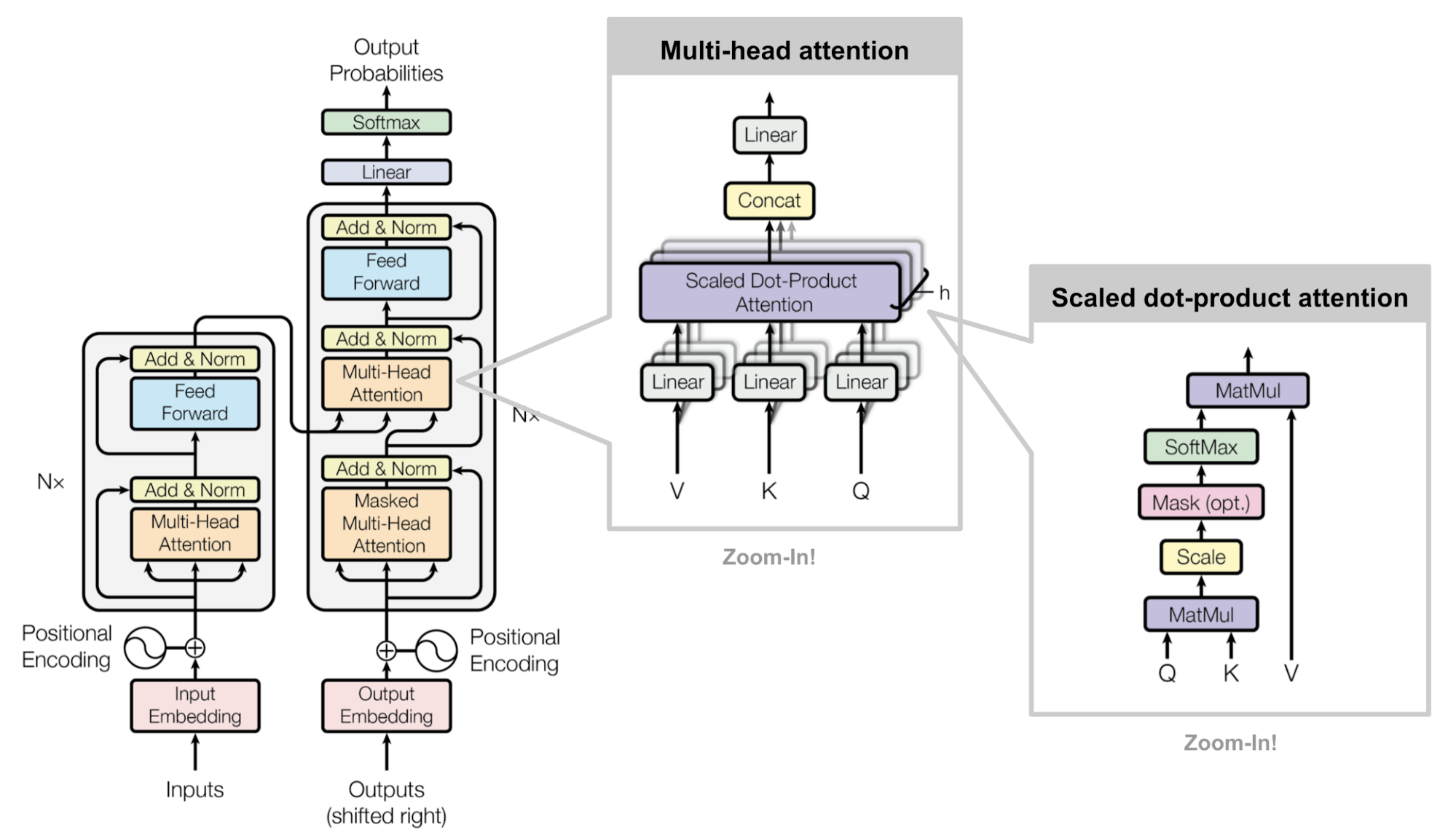Fig. 2. The architecture of the vanilla Transformer model. (Image source: Figure 17)

Positional Encoding

Because self-attention operation is permutation invariant, it is important to use proper positional encodingto provide order information to the model. The positional encoding $\mathbf{P} \in \mathbb{R}^{L \times d}$ has the same dimension as the input embedding, so it can be added on the input directly. The vanilla Transformer considered two types of encodings:

(1) Sinusoidal positional encoding is defined as follows, given the token position $i=1,\dots,L$ and the dimension $\delta=1,\dots,d$:

In this way each dimension of the positional encoding corresponds to a sinusoid of different wavelengths in different dimensions, from $2\pi$ to $10000 \cdot 2\pi$.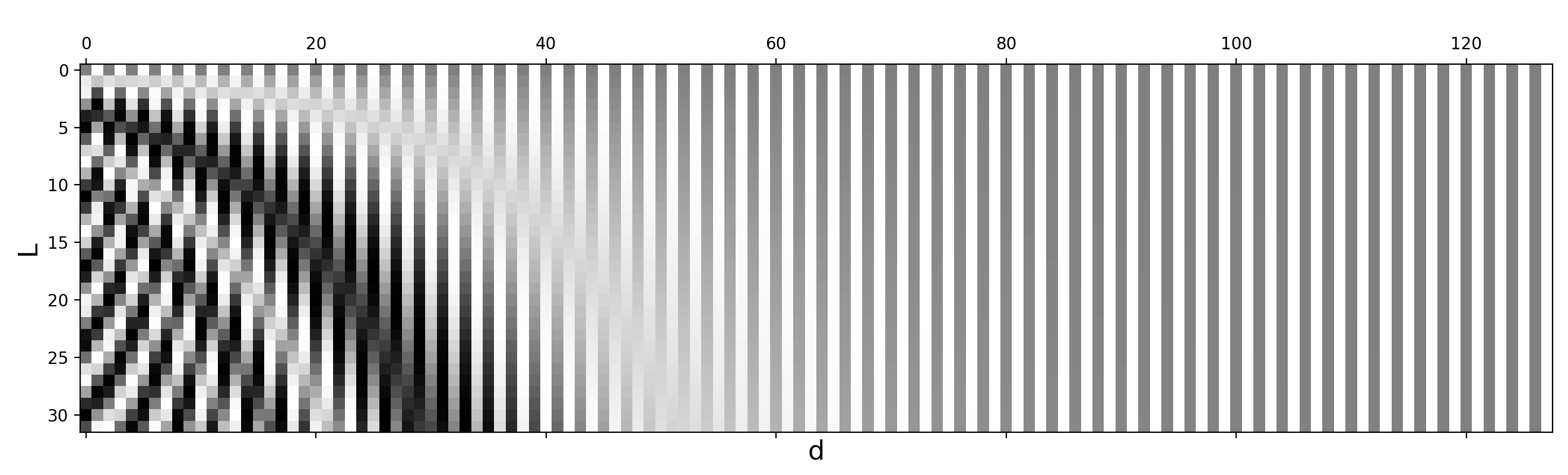Fig. 3. Sinusoidal positional encoding with $L=32$ and $d=128$. The value is between -1 (black) and 1 (white) and the value 0 is in gray.

(2) Learned positional encoding, as its name suggested, assigns each element with a learned column vector which encodes its absolute position (Gehring, et al. 2017).

Quick Follow-ups

Following the vanilla Transformer, Al-Rfou et al. (2018) added a set of auxiliary losses to enable training a deep Transformer model on character-level language modeling which outperformed LSTMs. Several types of auxiliary tasks are used:

• Instead of producing only one prediction at the sequence end, every immediate position is also asked to make a correct prediction, forcing the model to predict given smaller contexts (e.g. first couple tokens at the beginning of a context window).
• Each intermediate Transformer layer is used for making predictions as well. Lower layers are weighted to contribute less and less to the total loss as training progresses.
• Each position in the sequence can predict multiple targets, i.e. two or more predictions of the future tokens.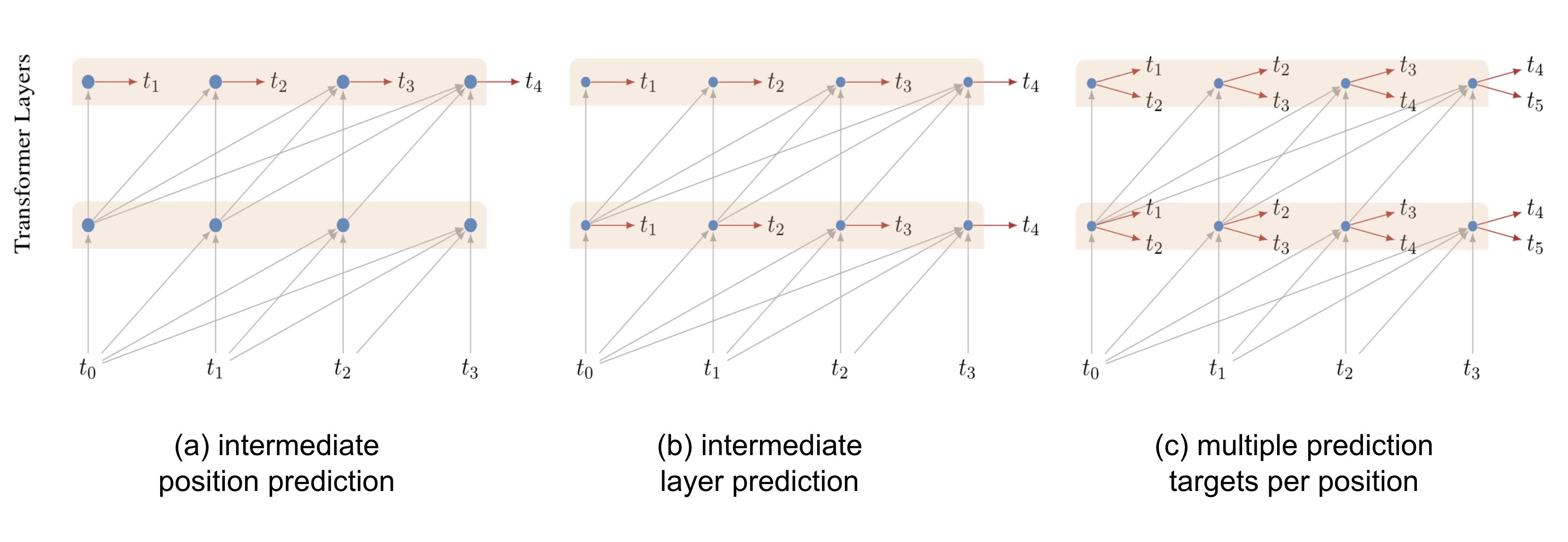Fig. 4. Auxiliary prediction tasks used in deep Transformer for character-level language modeling. (Image source: Al-Rfou et al. (2018))

Adaptive Computation Time (short for ACT; Graves, 2016) is a mechanism for dynamically deciding how many computational steps are needed in a recurrent neural network. Here is a cool tutorial on ACT from distill.pub.

Let’s say, we have a RNN model $\mathcal{R}$ composed of input weights $W_x$, a parametric state transition function $\mathcal{S}(.)$, a set of output weights $W_y$ and an output bias $b_y$. Given an input sequence $(x_1, \dots, x_L)$, the output sequence $(y_1, \dots, y_L)$ is computed by:

ACT enables the above RNN setup to perform a variable number of steps at each input element. Multiple computational steps lead to a sequence of intermediate states $(s_t^1, \dots, s_t^{N(t)})$ and outputs $(y_t^1, \dots, y_t^{N(t)})$ — they all share the same state transition function $\mathcal{S}(.)$, as well as the same output weights $W_x$ and bias $b_y$:

where $\delta_{n,1}$ is a binary flag indicating whether the input step has been incremented.

The number of steps $N(t)$ is determined by an extra sigmoidal halting unit $h$, with associated weight matrix $W_h$ and bias $b_h$, outputting a halting probability $p_t^n$ at immediate step $n$ for $t$-th input element:

In order to allow the computation to halt after a single step, ACT introduces a small constant $\epsilon$ (e.g. 0.01), so that whenever the cumulative probability goes above $1-\epsilon$, the computation stops.

where $M$ is an upper limit for the number of immediate steps allowed.

The final state and output are mean-field updates: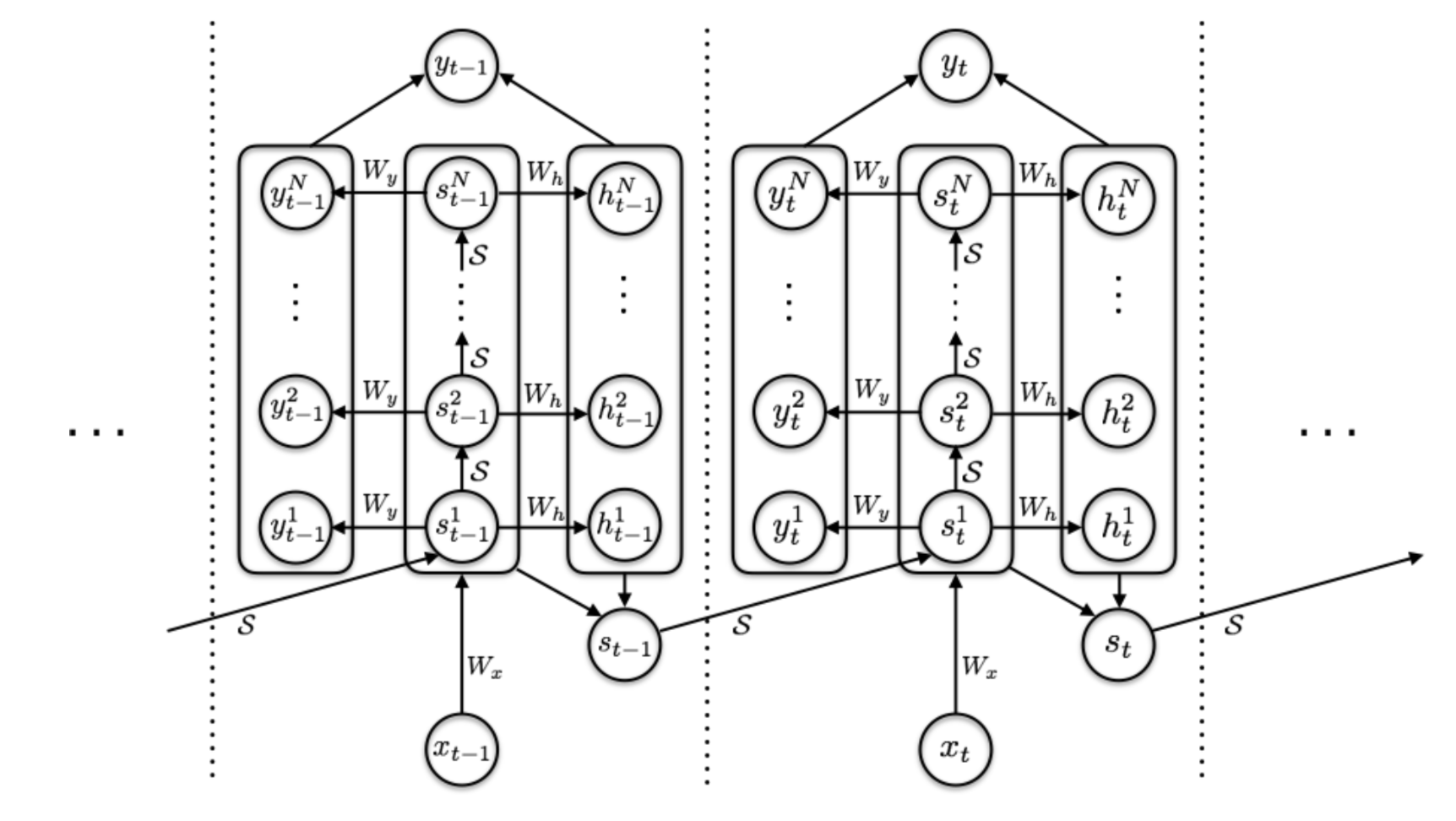Fig. 5. The computation graph of a RNN with ACT mechanism. (Image source: Graves, 2016)

To avoid unnecessary pondering over each input, ACT adds a ponder cost $\mathcal{P}(x) = \sum_{t=1}^L N(t) + R(t)$ in the loss function to encourage a smaller number of intermediate computational steps.

## Improved Attention Span

The goal of improving attention span is to make the context that can be used in self-attention longer, more efficient and flexible.

### Longer Attention Span (Transformer-XL)

The vanilla Transformer has a fixed and limited attention span. The model can only attend to other elements in the same segments during each update step and no information can flow across separated fixed-length segments.

This context segmentation causes several issues:

• The model cannot capture very long term dependencies.
• It is hard to predict the first few tokens in each segment given no or thin context.
• The evaluation is expensive. Whenever the segment is shifted to the right by one, the new segment is re-processed from scratch, although there are a lot of overlapped tokens.

Transformer-XL (Dai et al., 2019; “XL” means “extra long”) solves the context segmentation problem with two main modifications:

1. Reusing hidden states between segments.
2. Adopting a new positional encoding that is suitable for reused states.

Hidden State Reuse

The recurrent connection between segments is introduced into the model by continuously using the hidden states from the previous segments.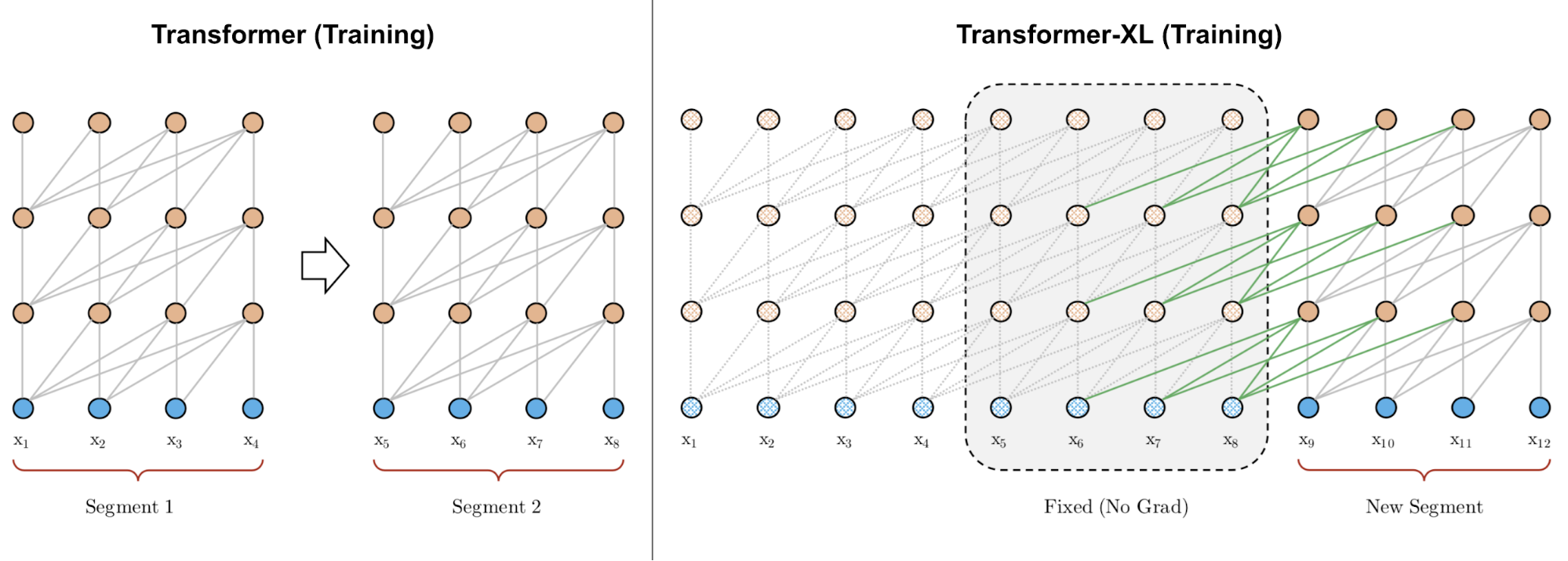Fig. 6. A comparison between the training phrase of vanilla Transformer & Transformer-XL with a segment length 4. (Image source: left part of Figure 2 in Dai et al., 2019).

Let’s label the hidden state of the $n$-th layer for the $(\tau + 1)$-th segment in the model as $\mathbf{h}_{\tau+1}^{(n)} \in \mathbb{R}^{L \times d}$. In addition to the hidden state of the last layer for the same segment $\mathbf{h}_{\tau+1}^{(n-1)}$, it also depends on the hidden state of the same layer for the previous segment $\mathbf{h}_{\tau}^{(n)}$. By incorporating information from the previous hidden states, the model extends the attention span much longer in the past, over multiple segments.

Note that both key and value rely on the extended hidden state, while the query only consumes hidden state at current step. The concatenation operation $[. \circ .]$ is along the sequence length dimension.

Relative Positional Encoding

In order to work with this new form of attention span, Transformer-XL proposed a new type of positional encoding. If using the same approach by vanilla Transformer and encoding the absolute position, the previous and current segments will be assigned with the same encoding, which is undesired.

To keep the positional information flow coherently across segments, Transformer-XL encodes the relative position instead, as it could be sufficient enough to know the position offset for making good predictions, i.e. $i-j$, between one key vector $\mathbf{k}_{\tau, j}$ and its query $\mathbf{q}_{\tau, i}$.

If omitting the scalar $1/\sqrt{d_k}$ and the normalizing term in softmax but including positional encodings, we can write the attention score between query at position $i$ and key at position $j$ as:

Transformer-XL reparameterizes the above four terms as follows:

• Replace $\mathbf{p}_j$ with relative positional encoding $\mathbf{r}_{i-j} \in \mathbf{R}^{d}$;
• Replace $\mathbf{p}_i\mathbf{W}^q$ with two trainable parameters $\mathbf{u}$ (for content) and $\mathbf{v}$ (for location) in two different terms;
• Split $\mathbf{W}^k$ into two matrices, $\mathbf{W}^k_E$ for content information and $\mathbf{W}^k_R$ for location information.

One key advantage of Transformer is the capability of capturing long-term dependencies. Depending on the context, the model may prefer to attend further sometime than others; or one attention head may had different attention pattern from the other. If the attention span could adapt its length flexibly and only attend further back when needed, it would help reduce both computation and memory cost to support longer maximum context size in the model.

This is the motivation for Adaptive Attention Span. Sukhbaatar, et al., (2019) proposed a self-attention mechanism that seeks an optimal attention span. They hypothesized that different attention heads might assign scores differently within the same context window (See Fig. 7) and thus the optimal span would be trained separately per head.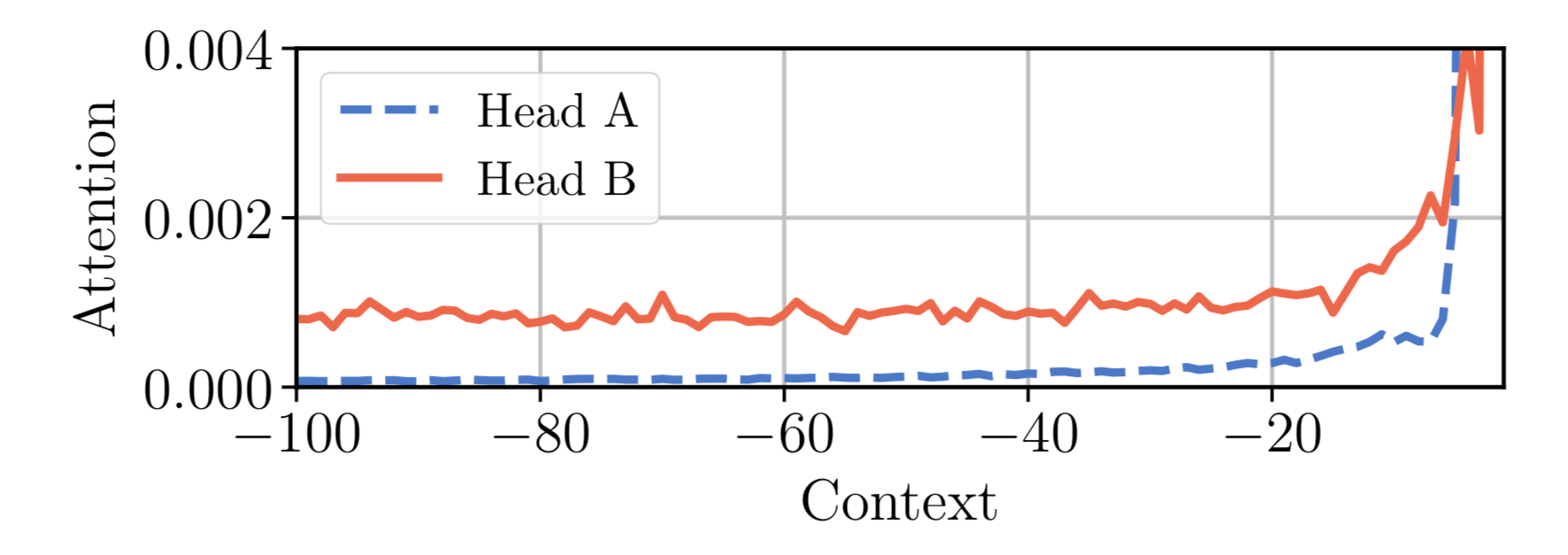Fig. 7. Two attention heads in the same model, A & B, assign attention differently within the same context window. Head A attends more to the recent tokens, while head B look further back into the past uniformly. (Image source: Sukhbaatar, et al. 2019)

Given the $i$-th token, we need to compute the attention weights between this token and other keys at positions $j \in S_i$, where $S_i$ defineds the $i$-th token’s context window.

A soft mask function $m_z$ is added to control for an effective adjustable attention span, which maps the distance between query and key into a [0, 1] value. $m_z$ is parameterized by $z \in [0, s]$ and $z$ is to be learned:

where $R$ is a hyper-parameter which defines the softness of $m_z$.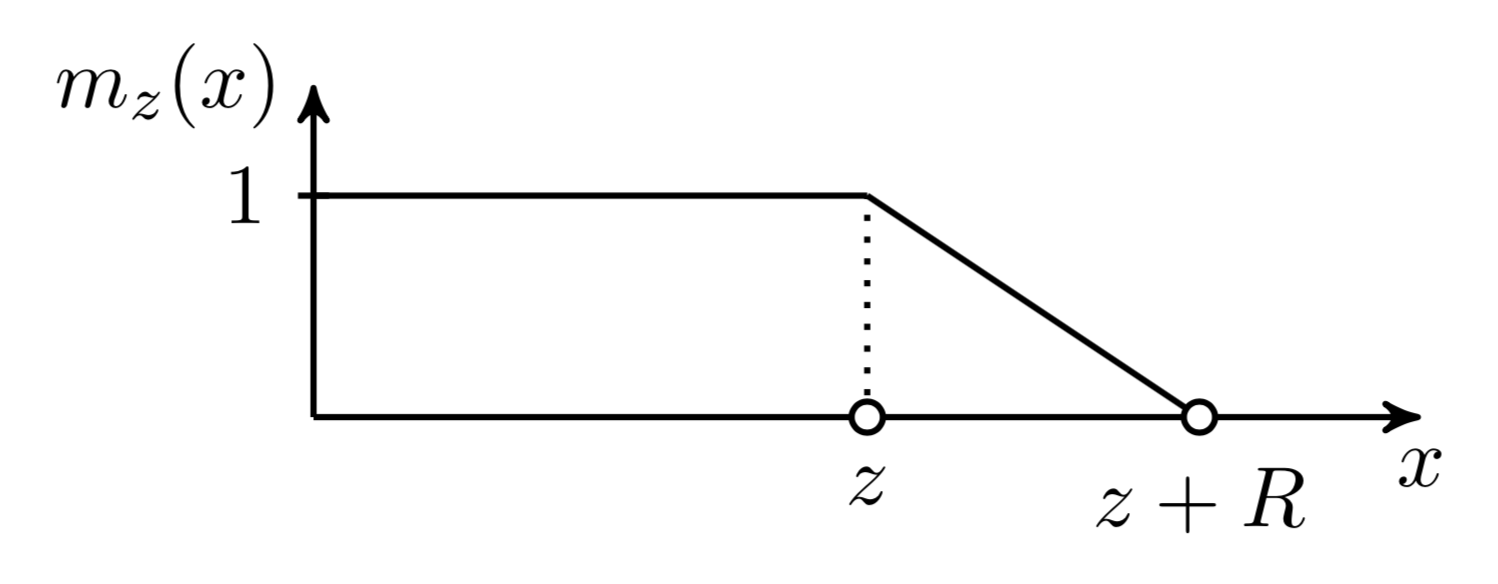Fig. 8. The soft masking function used in the adaptive attention span. (Image source: Sukhbaatar, et al. 2019.)

The soft mask function is applied to the softmax elements in the attention weights:

In the above equation, $z$ is differentiable so it is trained jointly with other parts of the model. Parameters $z^{(i)}, i=1, \dots, h$ are learned separately per head. Moreover, the loss function has an extra L1 penalty on $\sum_{i=1}^h z^{(i)}$.

Using Adaptive Computation Time, the approach can be further enhanced to have flexible attention span length, adaptive to the current input dynamically. The span parameter $z_t$ of an attention head at time $t$ is a sigmoidal function, $z_t = S \sigma(\mathbf{v} \cdot \mathbf{x}_t +b)$, where the vector $\mathbf{v}$ and the bias scalar $b$ are learned jointly with other parameters.

In the experiments of Transformer with adaptive attention span, Sukhbaatar, et al. (2019) found a general tendency that lower layers do not require very long attention spans, while a few attention heads in higher layers may use exceptionally long spans. Adaptive attention span also helps greatly reduce the number of FLOPS, especially in a big model with many attention layers and a large context length.

### Localized Attention Span (Image Transformer)

The original, also the most popular, use case for Transformer is to do language modeling. The text sequence is one-dimensional in a clearly defined chronological order and thus the attention span grows linearly with increased context size.

However, if we want to use Transformer on images, it is unclear how to define the scope of context or the order. Image Transformer (Parmer, et al 2018) embraces a formulation of image generation similar to sequence modeling within the Transformer framework. Additionally, Image Transformer restricts the self-attention span to only local neighborhoods, so that the model can scale up to process more images in parallel and keep the likelihood loss tractable.

The encoder-decoder architecture remains for image-conditioned generation:

• The encoder generates a contextualized, per-pixel-channel representation of the source image;
• The decoder auto-regressively generates an output image, one channel per pixel at each time step.

Let’s label the representation of the current pixel to be generated as the query $\mathbf{q}$. Other positions whose representations will be used for computing $\mathbf{q}$ are key vector $\mathbf{k}_1, \mathbf{k}_2, \dots$ and they together form a memory matrix $\mathbf{M}$. The scope of $\mathbf{M}$ defines the context window for pixel query $\mathbf{q}$.

Image Transformer introduced two types of localized $\mathbf{M}$, as illustrated below.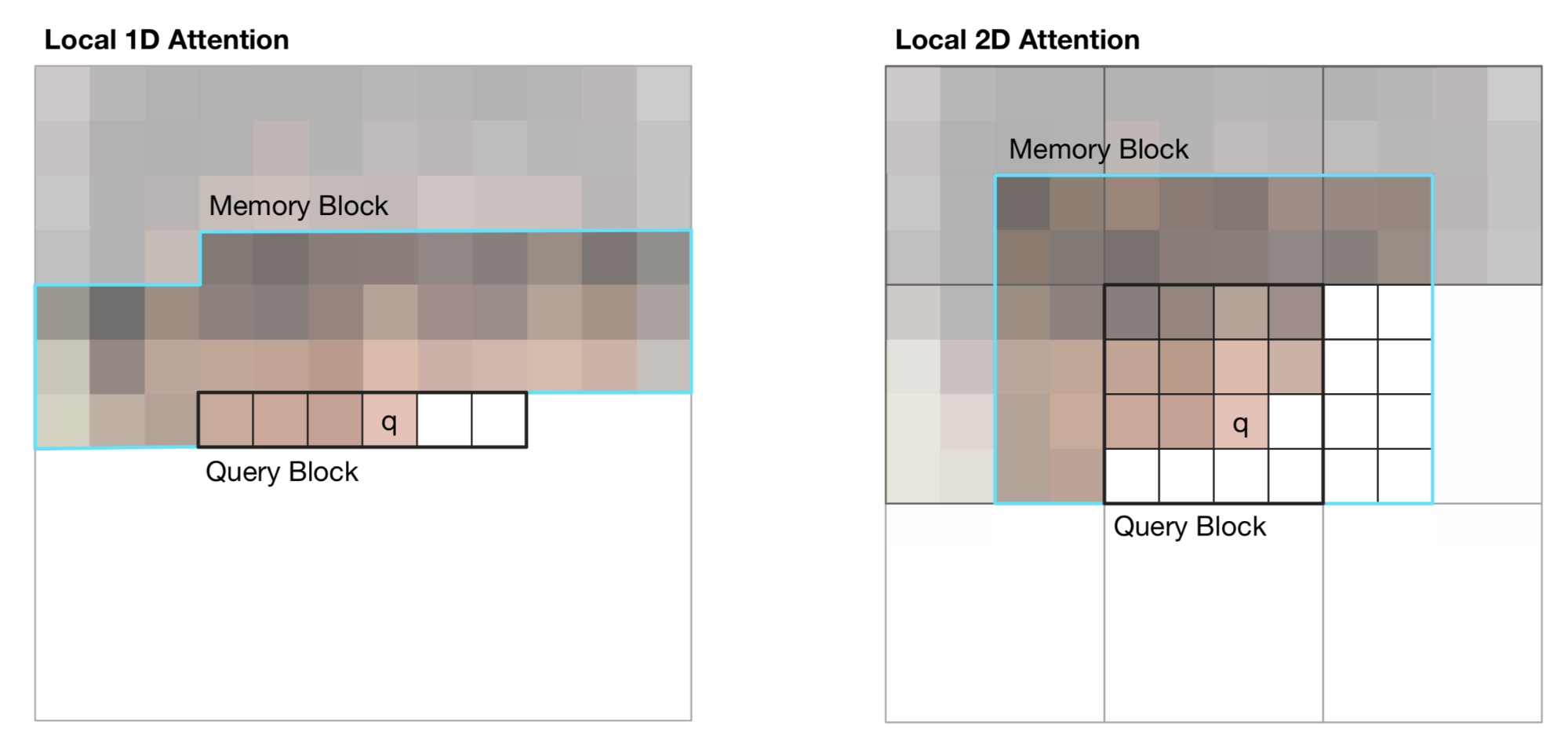Fig. 9. Illustration of 1D and 2D attention span for visual inputs in Image Transformer. The black line marks a query block and the cyan outlines the actual attention span for pixel q. (Image source: Figure 2 in Parmer et al, 2018)

(1) 1D Local Attention: The input image is flattened in the raster scanning order, that is, from left to right and top to bottom. The linearized image is then partitioned into non-overlapping query blocks. The context window consists of pixels in the same query block as $\mathbf{q}$ and a fixed number of additional pixels generated before this query block.

(2) 2D Local Attention: The image is partitioned into multiple non-overlapping rectangular query blocks. The query pixel can attend to all others in the same memory blocks. To make sure the pixel at the top-left corner can also have a valid context window, the memory block is extended to the top, left and right by a fixed amount, respectively.

## Less Time and Memory Cost

This section introduces several improvements made on Transformer to reduce the computation time and memory consumption.

### Sparse Attention Matrix Factorization (Sparse Transformers)

The compute and memory cost of the vanilla Transformer grows quadratically with sequence length and thus it is hard to be applied on very long sequences.

Sparse Transformer (Child et al., 2019) introduced factorized self-attention, through sparse matrix factorization, making it possible to train dense attention networks with hundreds of layers on sequence length up to 16,384, which would be infeasible on modern hardware otherwise.

Given a set of attention connectivity pattern $\mathcal{S} = \{S_1, \dots, S_n\}$, where each $S_i$ records a set of key positions that the $i$-th query vector attends to.

Note that although the size of $S_i$ is not fixed, $a(\mathbf{x}_i, S_i)$ is always of size $d_v$ and thus $\text{Attend}(\mathbf{X}, \mathcal{S}) \in \mathbb{R}^{L \times d_v}$.

In anto-regressive models, one attention span is defined as $S_i = \{j: j \leq i\}$ as it allows each token to attend to all the positions in the past.

In factorized self-attention, the set $S_i$ is decomposed into a tree of dependencies, such that for every pair of $(i, j)$ where $j \leq i$, there is a path connecting $i$ back to $j$ and $i$ can attend to $j$ either directly or indirectly.

Precisely, the set $S_i$ is divided into $p$ non-overlapping subsets, where the $m$-th subset is denoted as $A^{(m)}_i \subset S_i, m = 1,\dots, p$. Therefore the path between the output position $i$ and any $j$ has a maximum length $p + 1$. For example, if $(j, a, b, c, \dots, i)$ is a path of indices between $i$ and $j$, we would have $j \in A_a^{(1)}, a \in A_b^{(2)}, b \in A_c^{(3)}, \dots$, so on and so forth.

Sparse Factorized Attention

Sparse Transformer proposed two types of fractorized attention. It is easier to understand the concepts as illustrated in Fig. 10 with 2D image inputs as examples.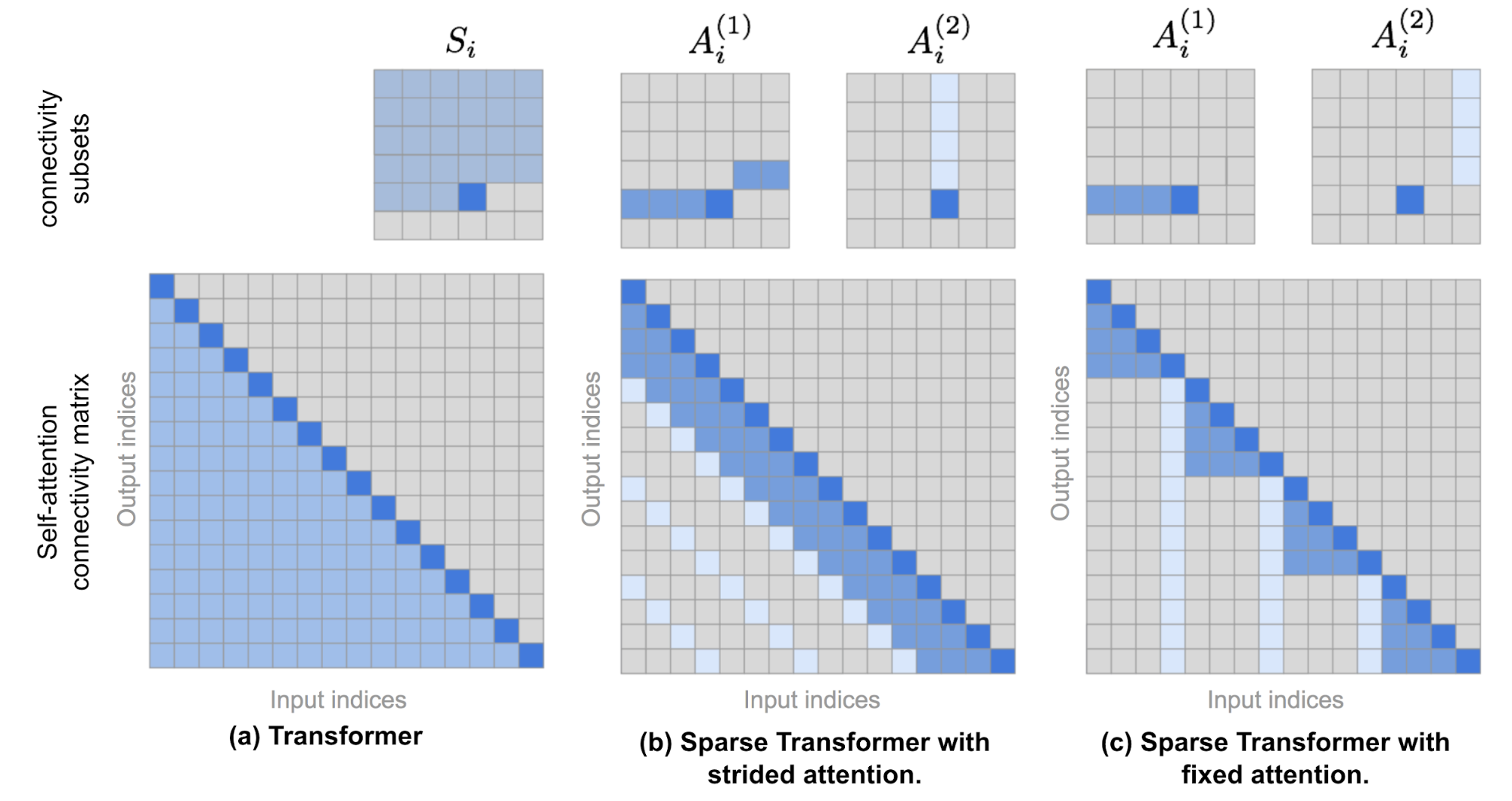Fig. 10. The top row illustrates the attention connectivity patterns in (a) Transformer, (b) Sparse Transformer with strided attention, and (c) Sparse Transformer with fixed attention. The bottom row contains corresponding self-attention connectivity matrices. Note that the top and bottom rows are not in the same scale. (Image source: Child et al., 2019 + a few of extra annotations.)

(1) Strided attention with stride $\ell \sim \sqrt{n}$. This works well with image data as the structure is aligned with strides. In the image case, each pixel would attend to all the previous $\ell$ pixels in the raster scanning order (naturally cover the entire width of the image) and then those pixels attend to others in the same column (defined by another attention connectivity subset).

(2) Fixed attention. A small set of tokens summarize previous locations and propagate that information to all future locations.

where $c$ is a hyperparameter. If $c=1$, it restricts the representation whereas many depend on a few positions. The paper chose $c\in \{ 8, 16, 32 \}$ for $\ell \in \{ 128, 256 \}$.

Use Factorized Self-Attention in Transformer

There are three ways to use sparse factorized attention patterns in Transformer architecture:

1. One attention type per residual block and then interleave them,
$\text{attention}(\mathbf{X}) = \text{Attend}(\mathbf{X}, A^{(n \mod p)}) \mathbf{W}^o$, where $n$ is the index of the current residual block.
2. Set up a single head which attends to locations that all the factorized heads attend to,
$\text{attention}(\mathbf{X}) = \text{Attend}(\mathbf{X}, \cup_{m=1}^p A^{(m)}) \mathbf{W}^o$.
3. Use a multi-head attention mechanism, but different from vanilla Transformer, each head might adopt a pattern presented above, 1 or 2. => This option often performs the best.

Sparse Transformer also proposed a set of changes so as to train the Transformer up to hundreds of layers, including gradient checkpointing, recomputing attention & FF layers during the backward pass, mixed precision training, efficient block-sparse implementation, etc. Please check the paper for more details.

### Locality-Sensitive Hashing (Reformer)

The improvements proposed by the Reformer model (Kitaev, et al. 2020) aim to solve the following pain points in Transformer:

• Memory in a model with $N$ layers is $N$-times larger than in a single-layer model because we need to store activations for back-propagation.
• The intermediate FF layers are often quite large.
• The attention matrix on sequences of length $L$ often requires $O(L^2)$ in both memory and time.

Reformer proposed two main changes:

1. Replace the dot-product attention with locality-sensitive hashing (LSH) attention, reducing the complexity from $O(L^2)$ to $O(L\log L)$.
2. Replace the standard residual blocks with reversible residual layers, which allows storing activations only once during training instead of $N$ times (i.e. proportional to the number of layers).

Locality-Sensitive Hashing Attention

In $\mathbf{Q} \mathbf{K}^\top$ part of the attention formula, we are only interested in the largest elements as only large elements contribute a lot after softmax. For each query $\mathbf{q}_i \in \mathbf{Q}$, we are looking for row vectors in $\mathbf{K}$ closest to $\mathbf{q}_i$. In order to find nearest neighbors quickly in high-dimensional space, Reformer incorporates Locality-Sensitive Hashing (LSH) into its attention mechanism.

A hashing scheme $x \mapsto h(x)$ is locality-sensitive if it preserves the distancing information between data points, such that close vectors obtain similar hashes while distant vectors have very different ones. The Reformer adopts a hashing scheme as such, given a fixed random matrix $\mathbf{R} \in \mathbb{R}^{d \times b/2}$ (where $b$ is a hyperparam), the hash function is $h(x) = \arg\max([xR; −xR])$.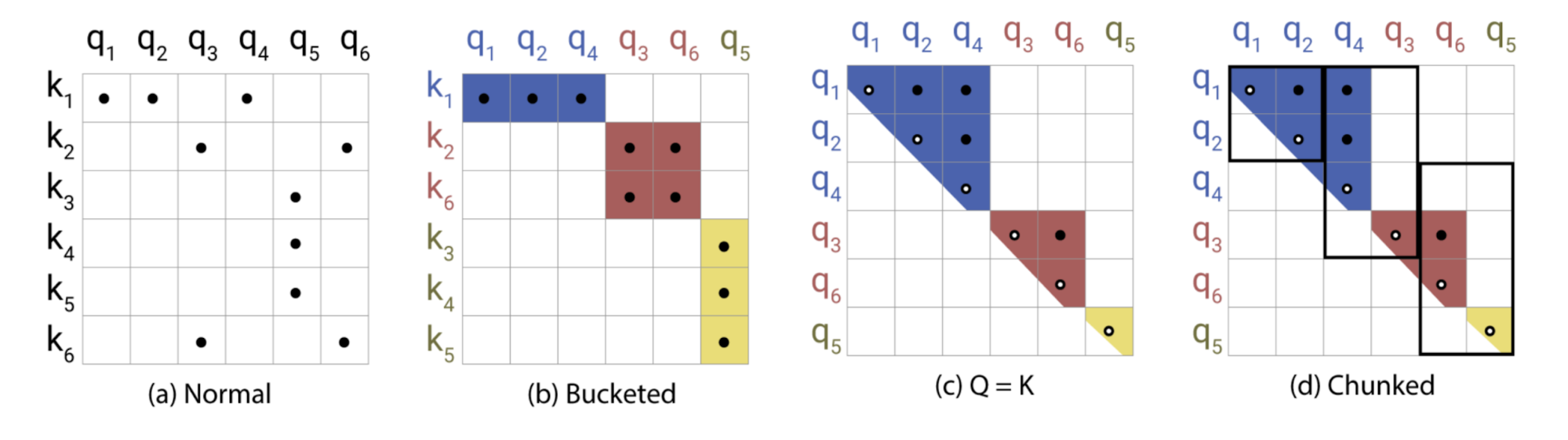Fig. 11. Illustration of Locality-Sensitive Hashing (LSH) attention. (Image source: right part of Figure 1 in Kitaev, et al. 2020).

In LSH attention, a query can only attend to positions in the same hashing bucket, $S_i = \{j: h(\mathbf{q}_i) = h(\mathbf{k}_j)\}$. It is carried out in the following process, as illustrated in Fig. 11:

• (a) The attention matrix for full attention is often sparse.
• (b) Using LSH, we can sort the keys and queries to be aligned according to their hash buckets.
• (c) Set $\mathbf{Q} = \mathbf{K}$ (precisely $\mathbf{k}_j = \mathbf{q}_j / \|\mathbf{q}_j\|$), so that there are equal numbers of keys and queries in one bucket, easier for batching. Interestingly, this “shared-QK” config does not affect the performance of the Transformer.
• (d) Apply batching where chunks of $m$ consecutive queries are grouped together.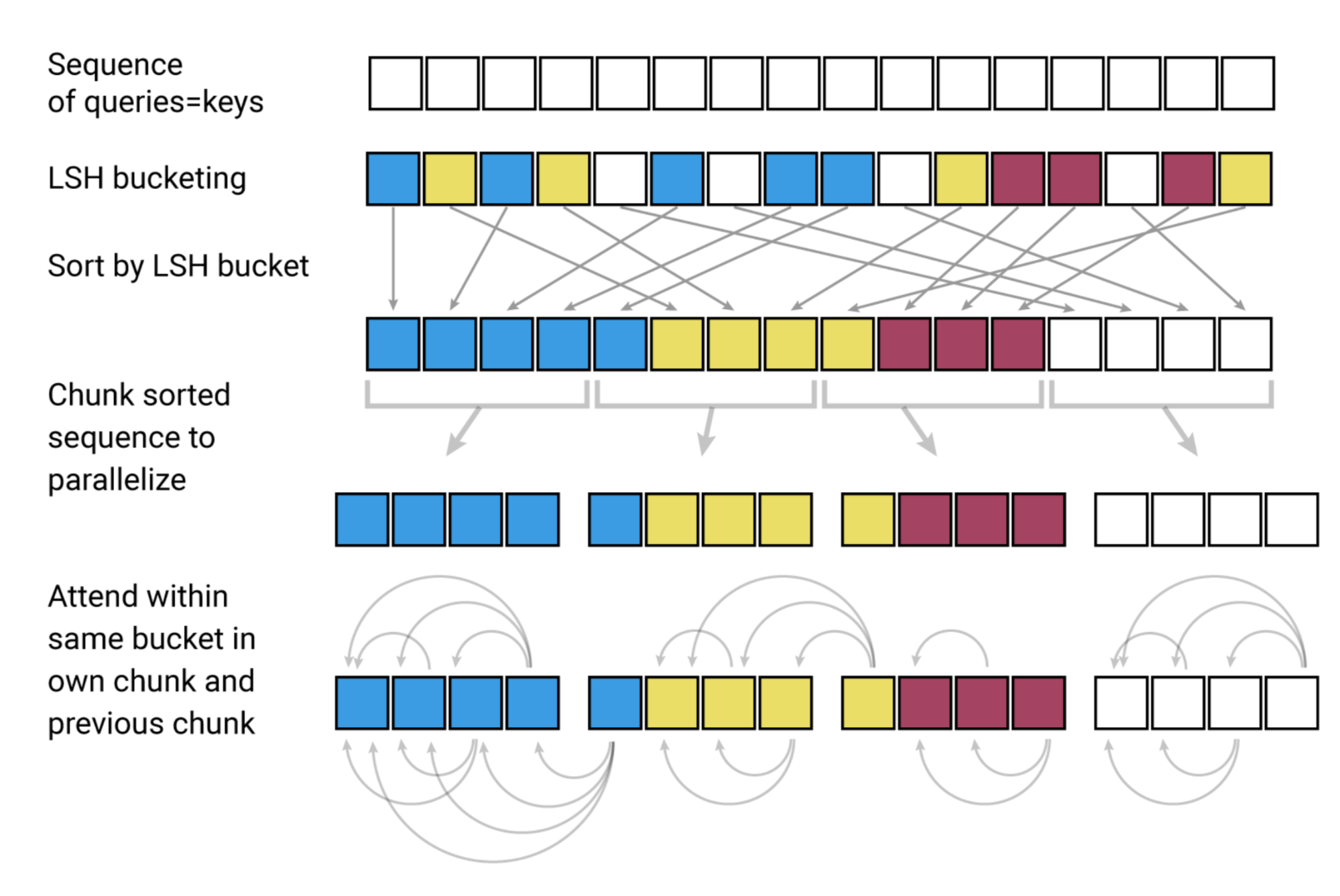Fig. 12. The LSH attention consists of 4 steps: bucketing, sorting, chunking, and attention computation. (Image source: left part of Figure 1 in Kitaev, et al. 2020).

Reversible Residual Network

Another improvement by Reformer is to use reversible residual layers (Gomez et al. 2017). The motivation for reversible residual network is to design the architecture in a way that activations at any given layer can be recovered from the activations at the following layer, using only the model parameters. Hence, we can save memory by recomputing the activation during backprop rather than storing all the activations.

Given a layer $x \mapsto y$, the normal residual layer does $y = x + F(x)$, but the reversible layer splits both input and output into pairs $(x_1, x_2) \mapsto (y_1, y_2)$ and then executes the following:

and reversing is easy:

Reformer applies the same idea to Transformer by combination attention ($F$) and feed-forward layers ($G$) within a reversible net block:

The memory can be further reduced by chunking the feed-forward computation: $Y_2 = [Y_2^{(1)}; \dots; Y_2^{(c)}] = [X_2^{(1)} + \text{FeedForward}(Y_1^{(1)}); \dots; X_2^{(c)} + \text{FeedForward}(Y_1^{(c)})]$

The resulting reversible Transformer does not need to store activation in every layer.

## Make it Recurrent (Universal Transformer)

The Universal Transformer (Dehghani, et al. 2019) combines self-attention in Transformer with the recurrent mechanism in RNN, aiming to benefit from both a long-term global receptive field of Transformer and learned inductive biases of RNN.

Rather than going through a fixed number of layers, Universal Transformer dynamically adjusts the number of steps using adaptive computation time. If we fix the number of steps, an Universal Transformer is equivalent to a multi-layer Transformer with shared parameters across layers.

On a high level, the universal transformer can be viewed as a recurrent function for learning the hidden state representation per token. The recurrent function evolves in parallel across token positions and the information between positions is shared through self-attention.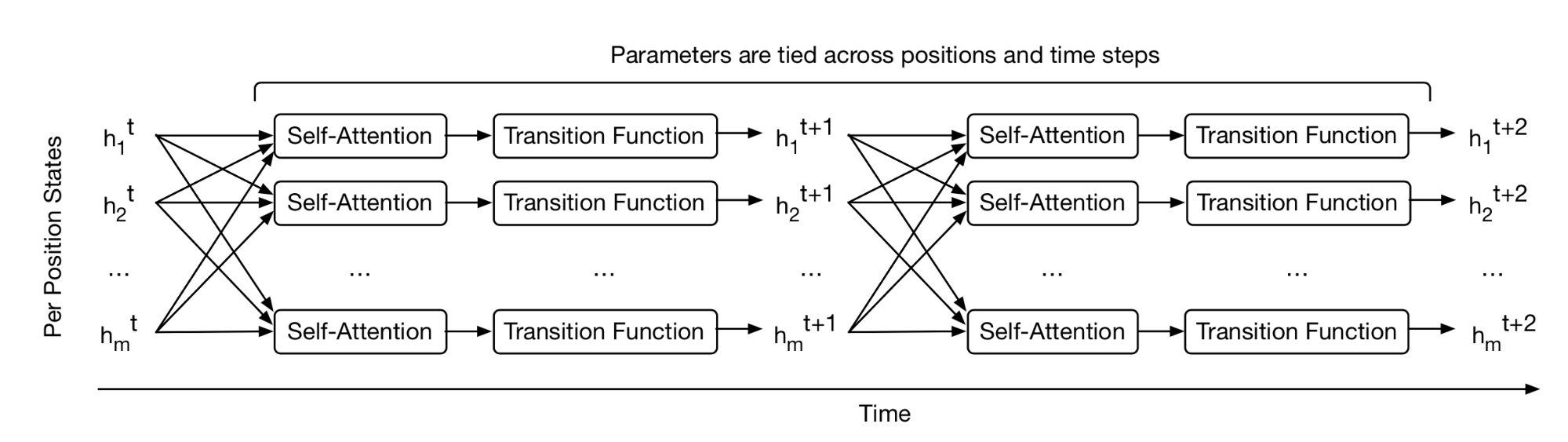Fig. 13. How the Universal Transformer refines a set of hidden state representations repeatedly for every position in parallel. (Image source: Figure 1 in Dehghani, et al. 2019).

Given an input sequence of length $L$, Universal Transformer iteratively updates the representation $\mathbf{H}^t \in \mathbb{R}^{L \times d}$ at step $t$ for an adjustable number of steps. At step 0, $\mathbf{H}^0$ is initialized to be same as the input embedding matrix. All the positions are processed in parallel in the multi-head self-attention mechanism and then go through a recurrent transition function.

where $\text{Transition}(.)$ is either a separable convolution or a fully-connected neural network that consists of two position-wise (i.e. applied to each row of $\mathbf{A}^t$ individually) affine transformation + one ReLU.

The positional encoding $\mathbf{P}^t$ uses sinusoidal position signal but with an additional time dimension: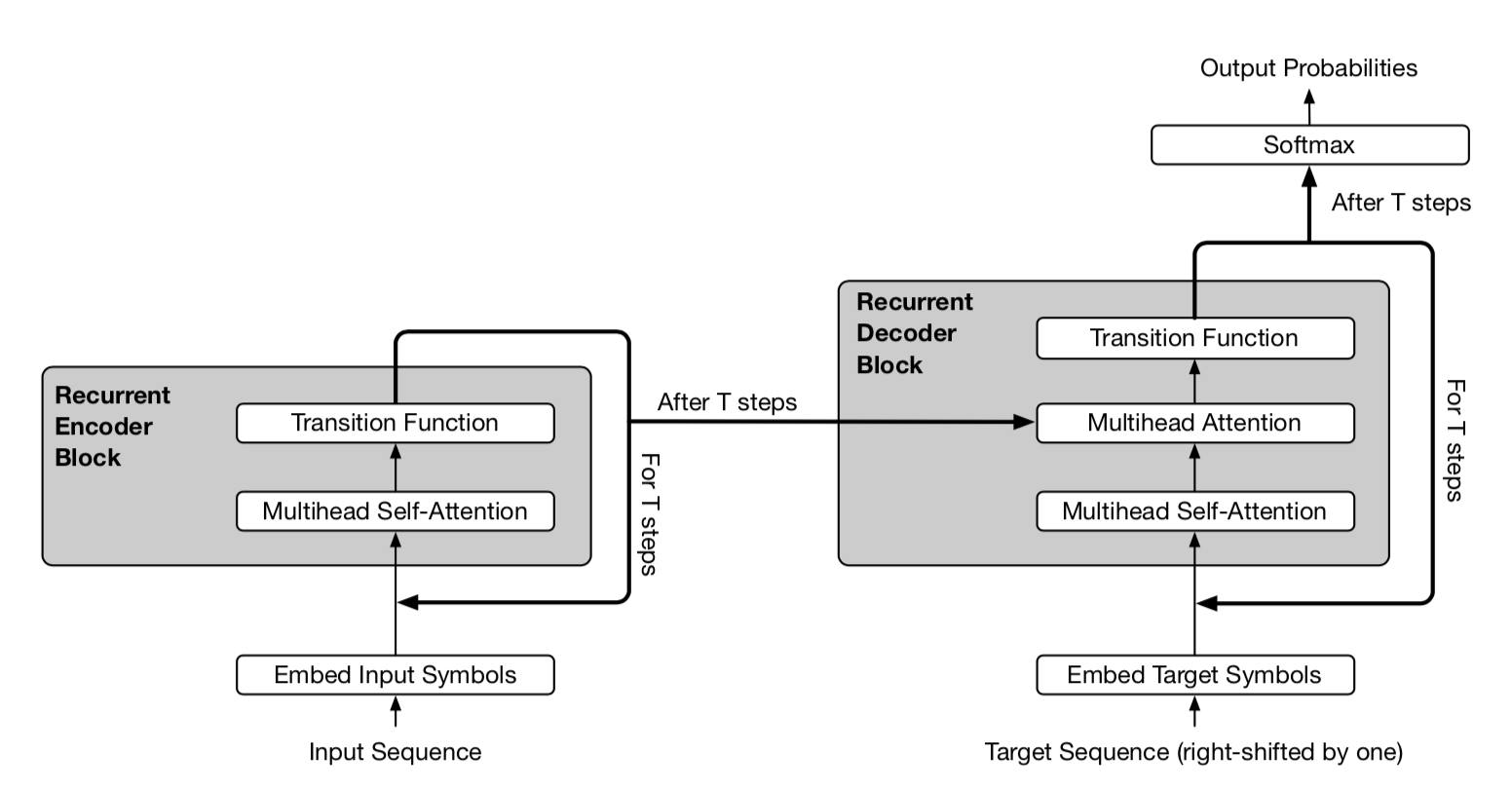Fig. 14. A simplified illustration of Universal Transformer. The encoder and decoder share the same basic recurrent structure. But the decoder also attends to final encoder representation $\mathbf{H}^T$. (Image source: Figure 2 in Dehghani, et al. 2019)

In the adaptive version of Universal Transformer, the number of recurrent steps $T$ is dynamically determined by ACT. Each position is equipped with a dynamic ACT halting mechanism. Once a per-token recurrent block halts, it stops taking more recurrent updates but simply copies the current value to the next step until all the blocks halt or until the model reaches a maximum step limit.

## Stabilization for RL (GTrXL)

The self-attention mechanism avoids compressing the whole past into a fixed-size hidden state and does not suffer from vanishing or exploding gradients as much as RNNs. Reinforcement Learning tasks can for sure benefit from these traits. However, it is quite difficult to train Transformer even in supervised learning, let alone in the RL context. It could be quite challenging to stabilize and train a LSTM agent by itself, after all.

The Gated Transformer-XL (GTrXL; Parisotto, et al. 2019) is one attempt to use Transformer for RL. GTrXL succeeded in stabilizing training with two changes on top of Transformer-XL:

1. The layer normalization is only applied on the input stream in a residual module, but NOT on the shortcut stream. A key benefit to this reordering is to allow the original input to flow from the first to last layer.
2. The residual connection is replaced with a GRU-style (Gated Recurrent Unit; Chung et al., 2014) gating mechanism.

The gating function parameters are explicitly initialized to be close to an identity map - this is why there is a $b_g$ term. A $b_g > 0$ greatly helps with the learning speedup.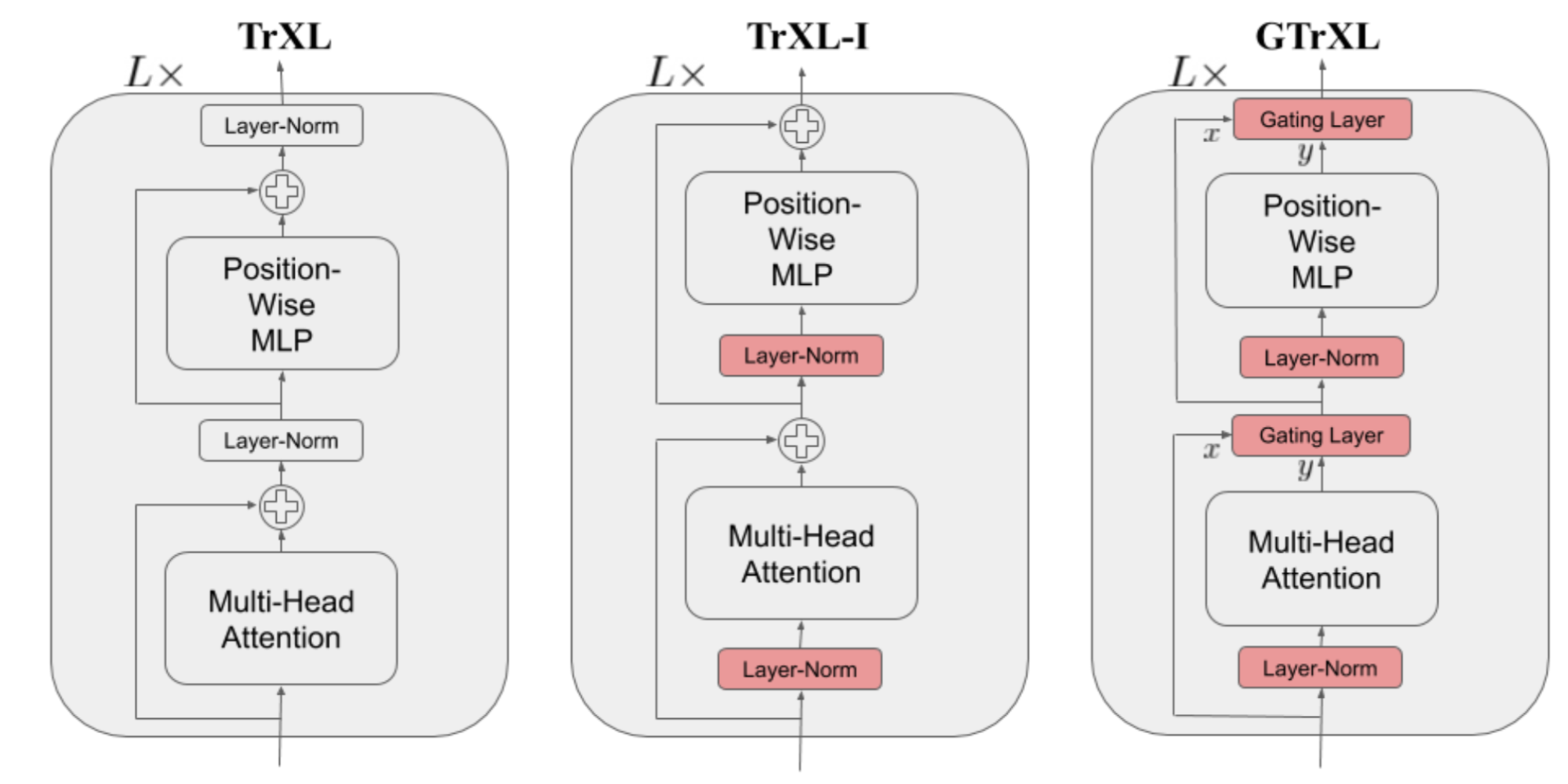Fig. 15. Comparison of the model architecture of Transformer-XL, Transformer-XL with the layer norm reordered, and Gated Transformer-XL. (Image source: Figure 1 in Parisotto, et al. 2019)

Cited as:

@article{weng2020transformer,
title   = "The Transformer Family",
author  = "Weng, Lilian",
journal = "lilianweng.github.io/lil-log",
year    = "2020",
url     = "https://lilianweng.github.io/lil-log/2020/03/27/the-transformer-family.html"
}


 Ashish Vaswani, et al. “Attention is all you need.” NIPS 2017.

 Rami Al-Rfou, et al. “Character-level language modeling with deeper self-attention.” AAAI 2019.

 Olah & Carter, “Attention and Augmented Recurrent Neural Networks”, Distill, 2016.

 Sainbayar Sukhbaatar, et al. “Adaptive Attention Span in Transformers”. ACL 2019.

 Rewon Child, et al. “Generating Long Sequences with Sparse Transformers” arXiv:1904.10509 (2019).

 Nikita Kitaev, et al. “Reformer: The Efficient Transformer” ICLR 2020.

 Alex Graves. (“Adaptive Computation Time for Recurrent Neural Networks”)[https://arxiv.org/abs/1603.08983]

 Niki Parmar, et al. “Image Transformer” ICML 2018.

 Zihang Dai, et al. “Transformer-XL: Attentive Language Models Beyond a Fixed-Length Context.” ACL 2019.

 Aidan N. Gomez, et al. “The Reversible Residual Network: Backpropagation Without Storing Activations” NIPS 2017.

 Mostafa Dehghani, et al. “Universal Transformers” ICLR 2019.

 Emilio Parisotto, et al. “Stabilizing Transformers for Reinforcement Learning” arXiv:1910.06764 (2019).